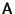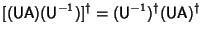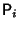## Hermitian Matrix

If a Matrix is Self-Adjoint, it is said to be a Hermitian matrix. Therefore, a Hermitian Matrix is defined as one for which(1)

wheredenotes the Adjoint Matrix. Hermitian Matrices have Real Eigenvalues with Orthogonal Eigenvectors. For Real Matrices, Hermitian is the same as symmetrical. Any Matrixwhich is not Hermitian can be expressed as the sum of two Hermitian matrices(2)

Letbe a Unitary Matrix andbe a Hermitian matrix. Then the Adjoint Matrix of a Similarity Transformation is(3)

The specific matrix(4)

whereare Pauli Spin Matrices, is sometimes called the'' Hermitian matrix.

Arfken, G. Hermitian Matrices, Unitary Matrices.'' §4.5 in Mathematical Methods for Physicists, 3rd ed. Orlando, FL: Academic Press, pp. 209-217, 1985.